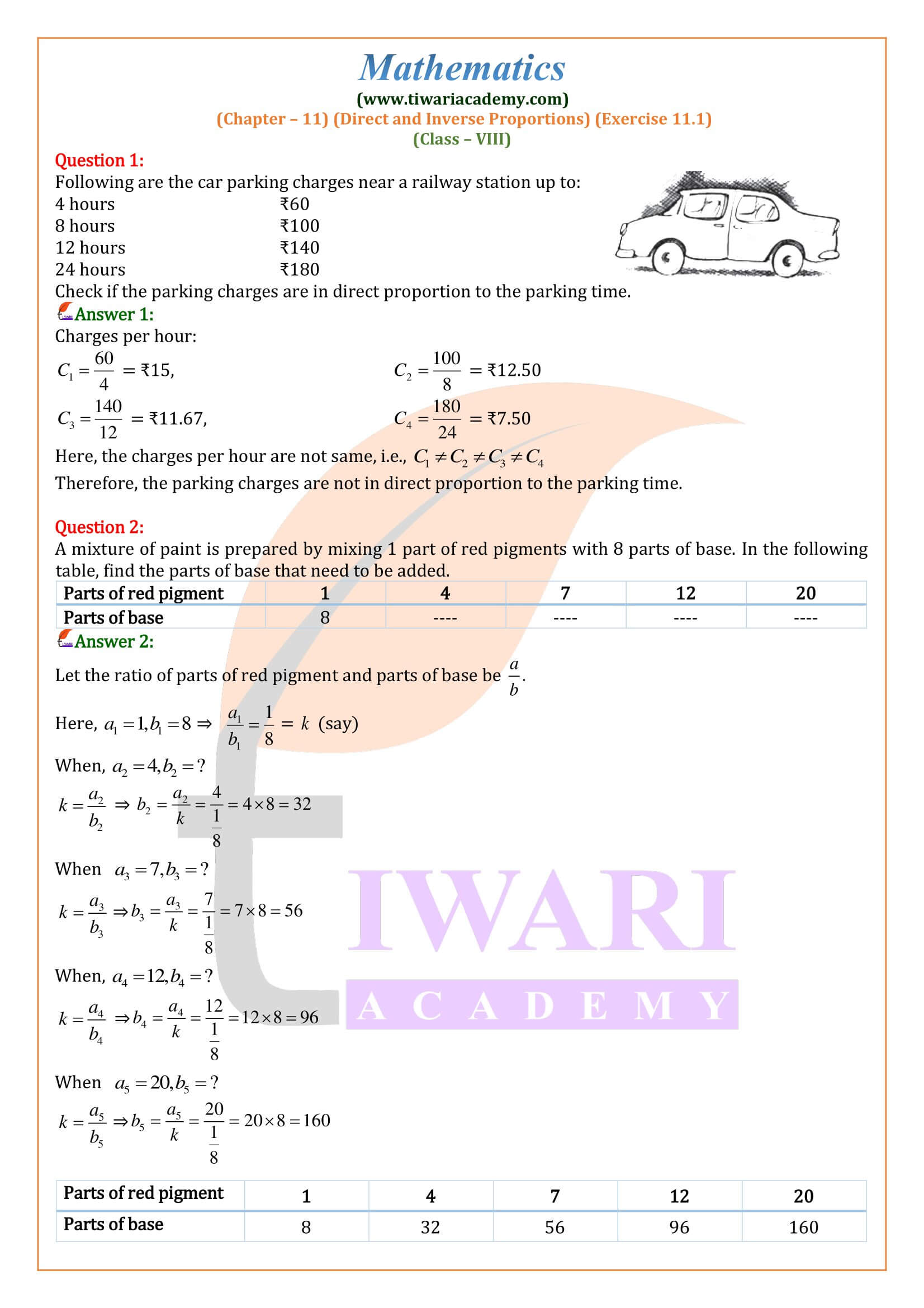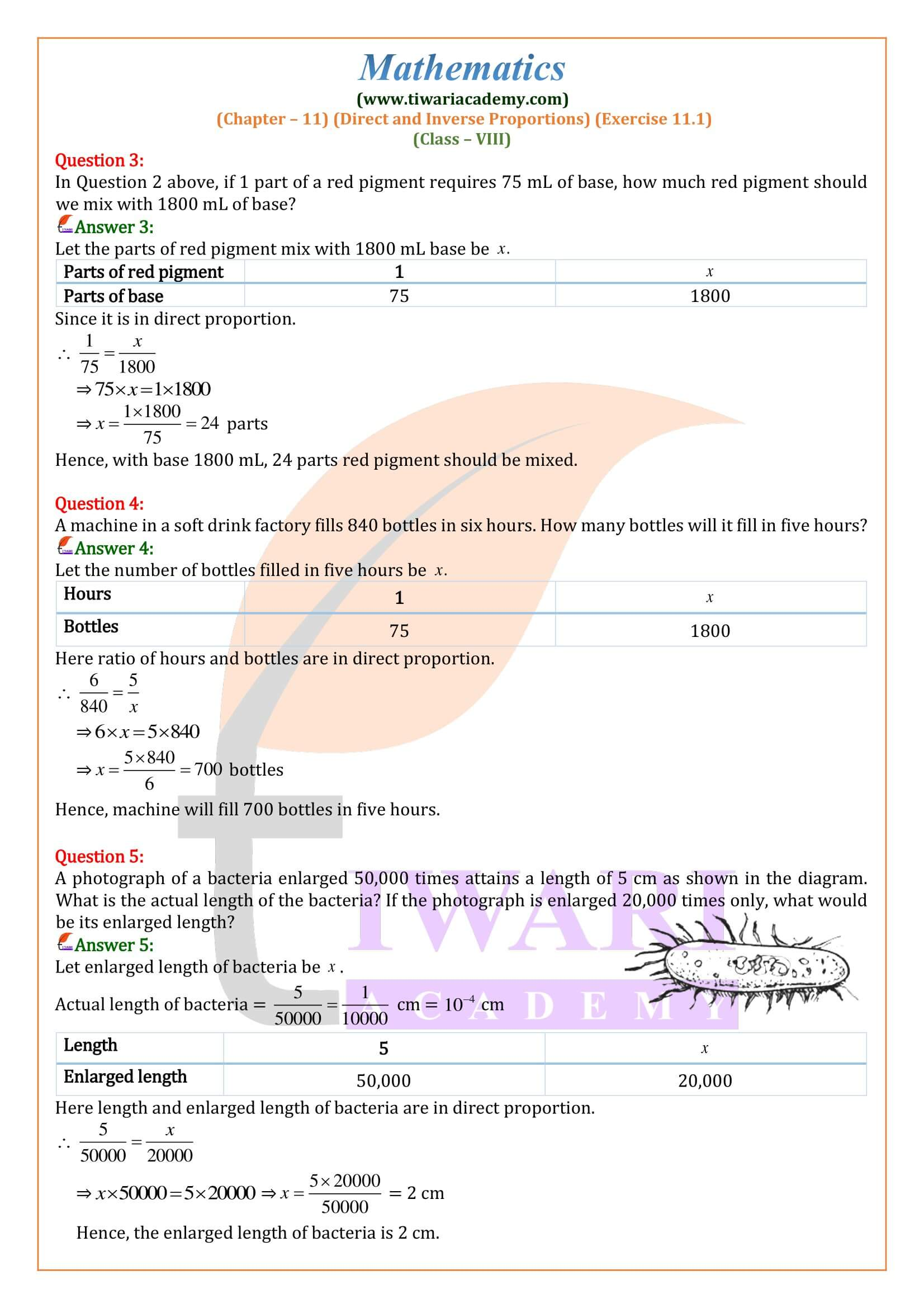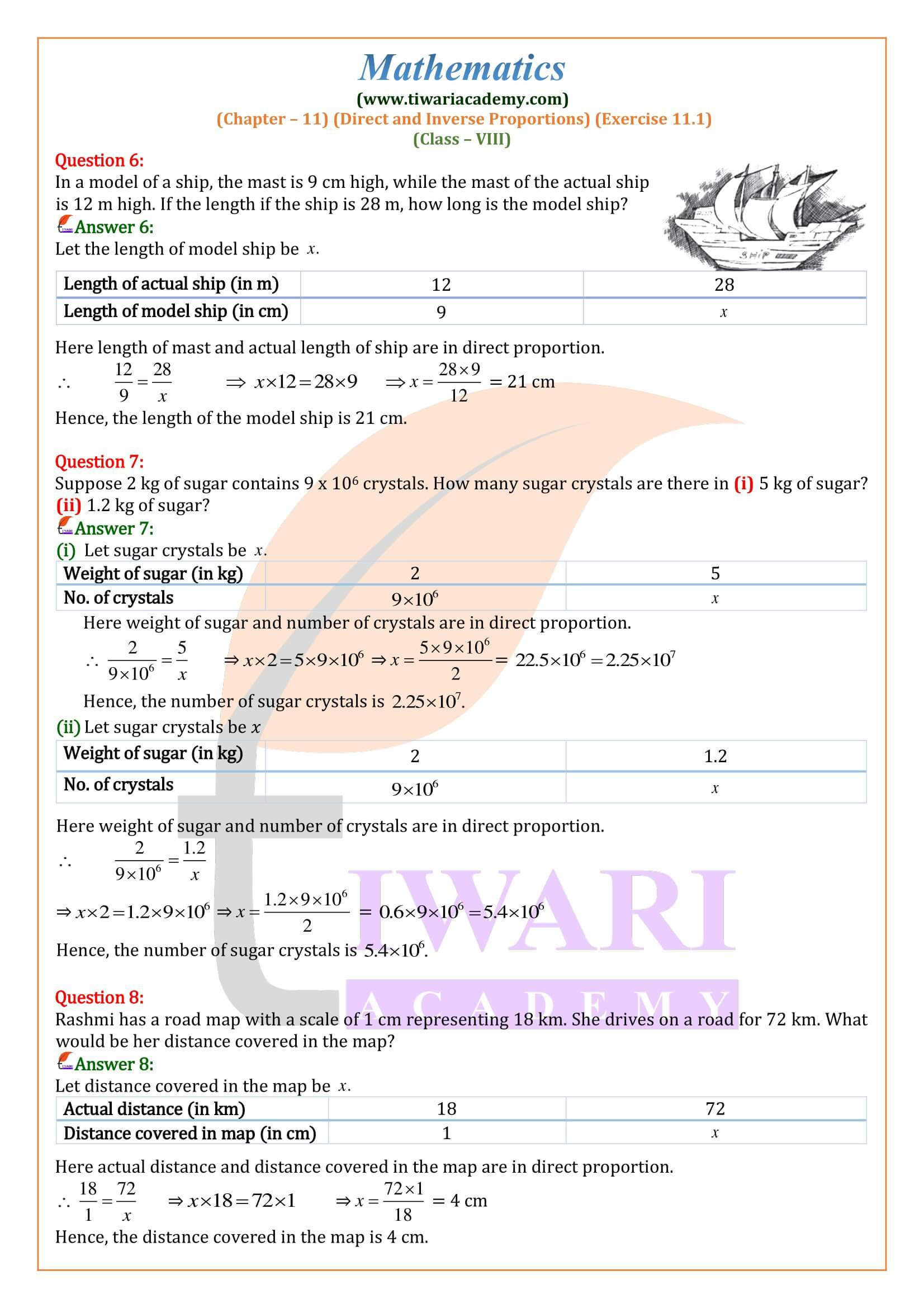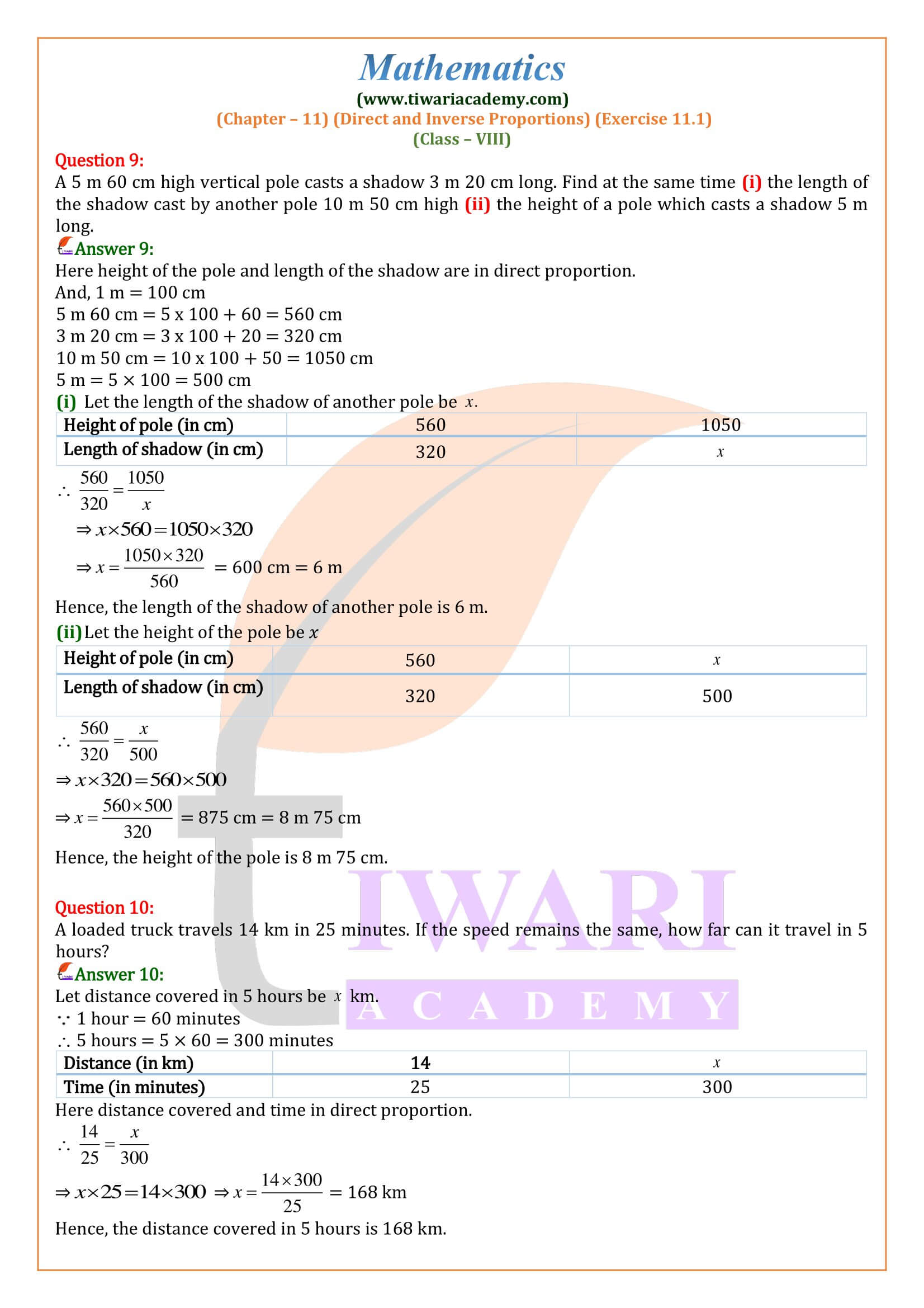NCERT Solutions for Class 8 Maths Chapter 11 Exercise 11.1 Direct and Inverse Proportions in Hindi and English Medium updated for CBSE 2023-24 exam. Get here the revised solutions of ex. 11.1 class 8 mathematics based on new syllabus and NCERT books issued for academic session 2023-24.

• ## 8th Maths Exercise 11.1 Solution in Hindi and English Medium

 Class: 8 Mathematics Chapter: 11 Exercise: 11.1 Topic Name: Direct and Inverse Proportions Content Type: PDF and Videos Solutions Academic Session: Session 2023-24 Medium: English and Hindi Medium

### Class 8 Maths Chapter 11 Exercise 11.1 Solution

Class VIII Mathematics NCERT chapter 11 Ex. 11.1 of Direct and Inverse Proportions in Hindi and English Medium for CBSE Exams 2023-24 free. All the questions of exercises are also solve in videos. Please take help of videos if any doubt in PDF solutions. In Class 8 Maths exercise 11.1 we will do the questions related to direct proportions and its application. If someone is facing problem to access the contents of Tiwari Academy, please contact us for help.

#### Variation

If two quantities depend on each other in such a way that the change in one results in a corresponding change in the other, then the two quantities are said to be in variation.
There are many situations in our daily life where the variation in one quantity brings a variation in the other.
Example:

1. More articles will cost more.
2. More is the money deposited in a bank, more is the interest earned in a fixed period,
3. More is the distance covered by a car, more is the petrol consumed by it.
4. More is the speed of a car, less is the time taken to cover a fixed distance.
5. More is the number of workers at a work, less is the time taken to complete the work.
##### Direct Proportion (or Direct Variation)

Two quantities x and y are said to be in direct proportion if whenever the value of x increases (or decreases), then the value of y increases (or decreases) in such a way that the ratio x/y remains constant.
Thus, x and y are in direct proportion, if x/y = k, where k is a constant, i.e.,
X1/y1 = x2/y2 = x3/y3 = ……= k
Example (i), (ii) and (iii) given above are the cases of direct proportion.
Remark: of y increases (or decreases) in such a way that the ratio remains constant. When x and y are in direct proportion, we also say that x and y have a direct variation.

A car covers 432 km in 36 litres of petrol. How much distance would it cover in 25 litres of petrol.
Let the required distance be x km. Then, we have:
Car covers 432 km in 36 liters, let, distance covered in 25 liters is x km then,
Clearly, less is the quantity of petrol consumed, less is the distance would it covered. So, it is a case of direct proportion.
36/432 = 25/x
Or, x = (432 x 25)/36 = 300 km
Hence, car covered 300 km. in 25 liters’ petrol.

How do you solve direct variations?
Direct variation problems are solved using the equation y = kx. In this case, you should use d for distance and t for time instead of x and y and notice how the word “square” changes the equation.

What is a real life example of direct variation?
There are many quantities present in our deal life which have direct and inverse relation.

1. The number of family members are directly expenditures.
2. The number of fruit in kg directly proportional to price of fruit.
3. The number of selling products directly proportional to profit.
4. The number of hours you work and the amount of your paycheck.
5. The amount of weight on a spring and the distance the spring will stretch.
6. The speed of a car and the distance traveled in a certain amount of time.

How do you define direct variation?
Direct variation describes a simple relationship between two variables. We say y varies directly with x (or as x, in some textbooks) if: y = kx, for some constant k, called the constant of variation or constant of proportionality.

### How many questions are there in exercise 11.1 of 8th Maths?

In exercise 11.1 of chapter 11 Direct and Inverse proportions from class 8 NCERT book have 10 questions based on the concept of proportion. These questions are easy and explained with 5 examples which can be used to solve without any difficulty.

### What are the basic skills required to study class 8 Maths Exercise 11.1?

The concept of direct proportion in 8th Maths is that when one quantity increases, the other quantity also varies in the same ratio. That is if one quantity is double than the other, then, the other should also be increased by two times or halved. Apart from this, the basic multiplication and division skills are important.

### Is exercise 11.1 of class 8 Maths easy?

All the questions in exercise 11.1 of chapter 11 belongs to direct and inverse proportion. NCERT solutions for Maths class 8 ex 11.1 are based on the direct proportion principle. Only the types and statements of the questions are changed. Questions required people to use their reasoning skills to perform well. However, questions are straight calculation based also.Last Edited: April 17, 2023